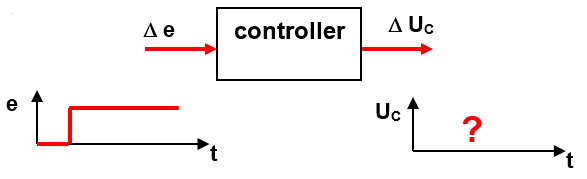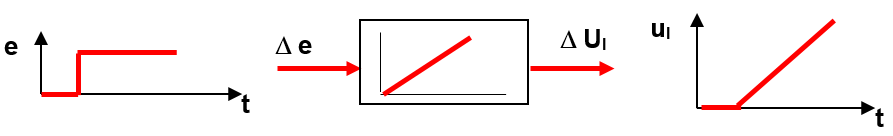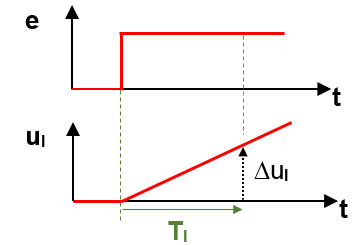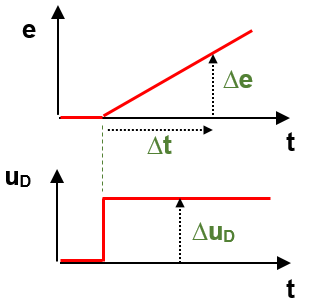# Analog controller

### Analog controllers

Examples of analog controls: speed control, positioning control, torque control, voltage control, etc. What do all these controls have in common? They control a fast reacting system!Block diagram of a PID control

Let us explain the function of a PID controller in this video:step response controller

Analyze the control behavior

Principle: A voltage jump of ideally 1V is applied to the input of the controller. The parameters of the controller are determined by means of the step response. We regard the P, I and D shares on their own:

.

P-controller or proportional controller
A P-controller has an output signal u(t), that is proportional to the control error (u ~ e) without delay. As soon as the control error returns to the value 0, this controller does not have an output signal u any more.step response P-controller

Determine the parameter proportional gain factor Kp:  Kp  =   Δ u / Δe

Although a P-controller reacts immediately, a pure P-control has a permanent control deviation!

I-Controller or Integral-controller

The integral part is used for optimization because it can reduce a control deviation to zero. As you can see, the Integral force increases steadily as long as there is still a control deviation. When the control deviation is zero, the I-force remains at its constant value and reduces again only by a control deviation with opposite sign.step response Integral-controller

Determine of the parameters for the I-force:

Since the output signal of the I-force constantly changes during a control deviation, you would relate the slope of the I-force to the input quantity e:

Integral gain K :     K=   (Δ u / Δt) / Δ e  =    (u – u0) / (Δt  * Δe)

The unit of this integral gain KI is 1/s. Because this parameter is hard to understand for the user you would use the so-called Integral time, which represents the reciprocal:Integral-time I-controller

Integral time TI  =    1 /  K    =    (Δ t * Δe ) / Δu           (without regarding the sign)

To tell it in a few words: TI is the time until the output equals the level of the input signal.

.

.

D-controller or Differential controller

The differential-part also serves for optimization. With its force, the controller reacts to a change of a control deviation very quickly. It also dampens the oscillation of the control.step response D-controller

The D controller reacts to a change in the control deviation! Problem: No parameters can be derived from the step response of a D controller. Trick: As input signal we use a ramp:Determine parameter of a D-controller

Derivative gain KD

K    Δ u _ _     =   Δ u *  Δt
.          Δ e /  Δt                Δe

.

..

.

.

Exercise: Step response of a PI-Controller

Empirically, you have set the PID controller as best as possible with the following parameters:  KP = 1.5, Ti = 0.002 s

A voltage jump of 1 volt is applied to the input of the controller. Complete the step response of the P- and I-force as well as the combined PI controller on the solution sheet.Exercise Step-response PI-controller